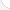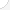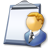Electrical engineersDescription: Design, develop, test, or supervise the manufacturing and installation of electrical equipment, components, or systems for commercial, industrial, military, or scientific use.Source: Bureau of Labor Statistics, U.S. Department of Labor, Occupational Outlook Handbook, 2008-09 Edition at http://www.bls.gov/OCO/Complete Job Profile: http://www.bls.gov/oco/ocos027.htmSalary: \$50,001 or more per yearComments:

There are 53 math topics Electrical engineers need to know.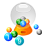Basic Math / Algebra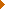FractionsDecimalsRatio and ProportionPercentCustomary MeasurementMetric MeasurementMeasurement ConversionBasic ProbabilityBasic StatisticsStatistical GraphingPowers and RootsOther Number BasesNegative NumbersScientific NotationBasic Problem Solving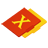First-Year AlgebraUsing FormulasLinear EquationsOperations with PolynomialsFactoring PolynomialsCoordinate Graphing 2DLinear SystemsQuadratic EquationsAlgebraic Representation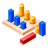GeometryBasic TerminologyAngle MeasurementPythagorean TheoremRight Triangle TrigonometryAreaVolumeMake/Use 3D Drawings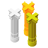Second-Year Algebra / TrigonometryFunctionsVariationImaginary NumbersPolynomial EquationsLogarithmsCoordinate Graphing 3DAdvanced ProbabilityAdvanced StatisticsNon-Linear SystemsTrigonometric/Circular FunctionsGraphs of Trigonometric FunctionsPolar Coordinates/GraphsVectors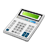Other TopicsCalculus and Higher MathBasic Calculator UseScientific Calculator UseComputer UseComputer ProgrammingGroup Problem SolvingMental MathInductive/Deductive ReasoningMath CommunicationsMathematical Modeling